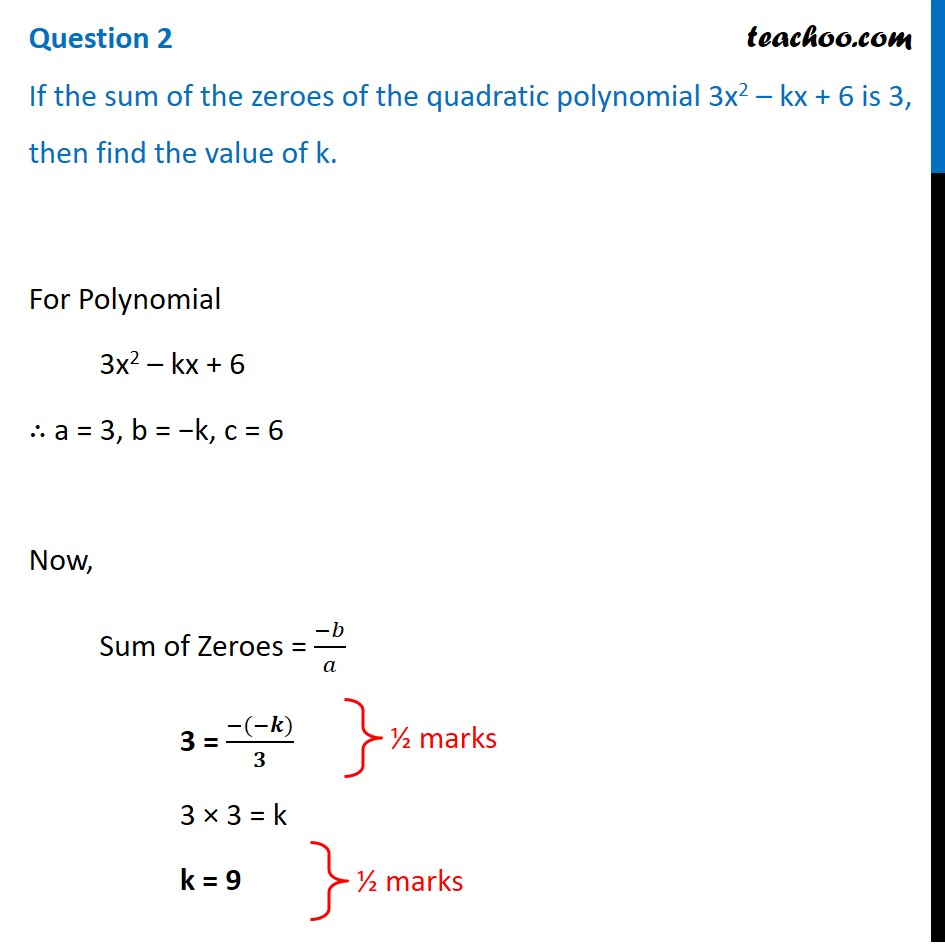CBSE Class 10 Sample Paper for 2021 Boards - Maths Standard

Class 10
Solutions of Sample Papers for Class 10 Boards

## If the sum of the zeroes of the quadratic polynomial 3x 2 – kx + 6 is 3, then find the value of k.Learn in your speed, with individual attention - Teachoo Maths 1-on-1 Class

### Transcript

Question 2 If the sum of the zeroes of the quadratic polynomial 3x2 – kx + 6 is 3, then find the value of k. For Polynomial 3x2 – kx + 6 ∴ a = 3, b = −k, c = 6 Now, Sum of Zeroes = (−𝑏)/𝑎 3 = (−(−𝒌))/𝟑 3 × 3 = k k = 9